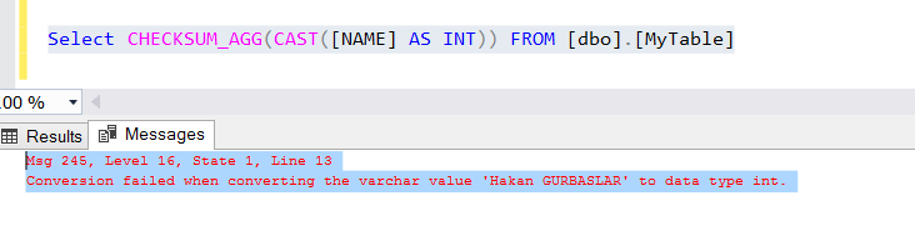Monday , September 25 2023

# CHECKSUM_AGG Function in SQL Server(TSQL)

Creates a checksum value of a data set. You can use it to check if data in a column is being modified. accepts int data type as a parameter and returns int value as a result.

Let’s make examples for a better understanding of CHECKSUM_AGG Function.

Example:

First, we create a table with the help of the following script and add a few records into this table.

We calculate the checksum of the data in the Age column with the CHECKSUM_AGG function as follows.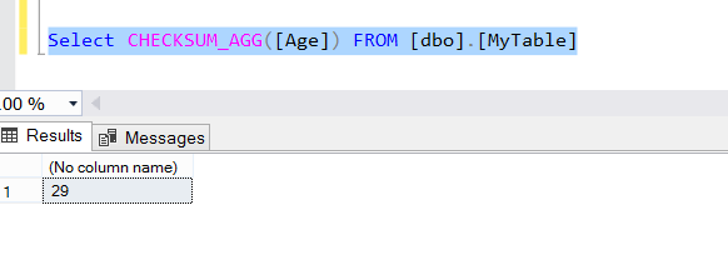Perform an update in the Age column as follows and recalculate the checksum value.

As you can see, the checksum value has changed.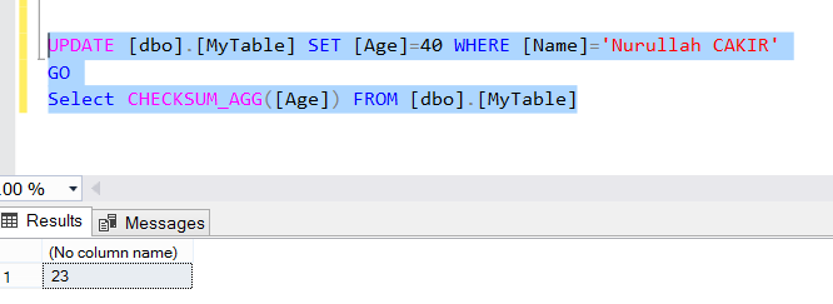Update the data to its old value and calculate the checksum again.

As you can see, its the first calculated checksum value.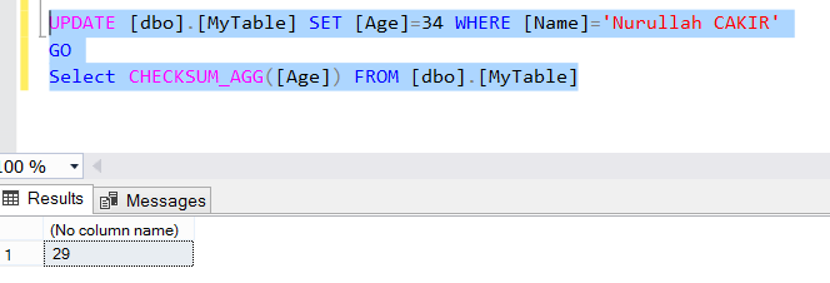When we run the checksum function in a column of the string(varchar,nvarchar etc) data type, we receive the error as follows.

Msg 8117, Level 16, State 1, Line 13

Operand data type varchar is invalid for checksum_agg operator.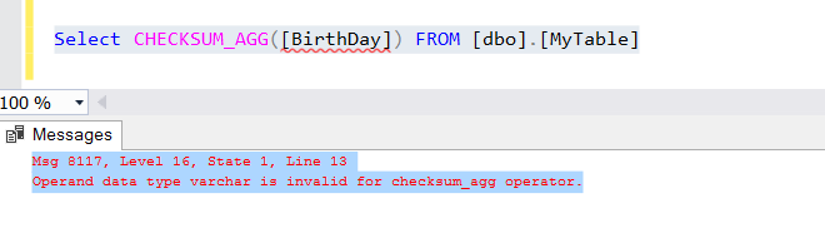We can use this function without error for string data type as follows.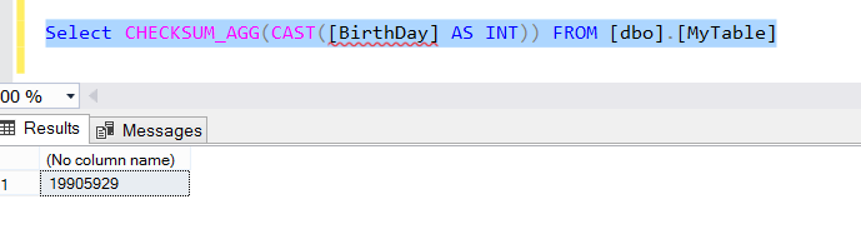Of course, we cannot convert each data to the INT data type with CAST. When we try to perform the same operation for the [Name] column, we get the following error.

Msg 245, Level 16, State 1, Line 13

Conversion failed when converting the varchar value ” to data type int.